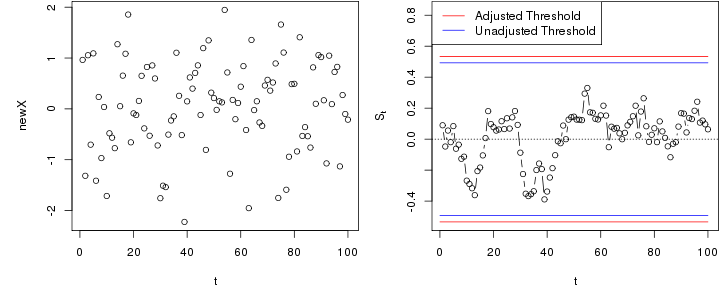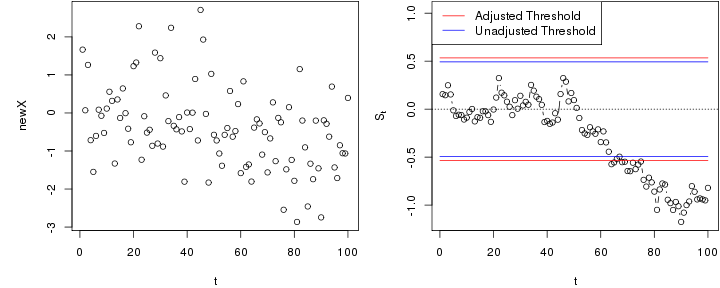# EWMA chart with estimated in-control state

## Using normality assumptions

The following is an illustration for EWMA charts, assuming that all observations are normally distributed.

Based on $$n$$ past in-control observations $$X_{-n},\dots,X_{-1}$$, the in-control mean and standard deviation can be estimated by the sample mean, $$\hat \mu$$, and sample standard deviation, $$\hat \sigma$$. For new observations $$X_1,X_2,\dots$$, an EWMA chart based on these estimated parameters is defined by $M_0=0, \quad M_t=\lambda \frac{X_t-\hat \mu}{\hat \sigma}+(1-\lambda) M_{t-1}$

The following generates a data set of past observations (replace this with your observed past data).

X <-  rnorm(250)


Next, we initialise the chart and compute the estimates needed for running the chart - in this case $$\hat \mu$$ and $$\hat \sigma$$.

library(spcadjust)
chart <- new("SPCEWMA",model=SPCModelNormal(Delta=0),lambda=0.1);
xihat <- xiofdata(chart,X)
str(xihat)

## List of 3
##  $mu: num 0.0251 ##$ sd: num 1.05
##  \$ m : int 250


## Calibrating the chart to a given average run length (ARL)

We now compute a threshold that with roughly 90% probability results in an average run length of at least 100 in control. This is based on parametric resampling assuming normality of the observations.

cal <- SPCproperty(data=X,nrep=50,
property="calARL",chart=chart,params=list(target=100),quiet=TRUE)
cal

## 90 % CI: A threshold of +/- 0.5342 gives an in-control ARL of at
##   least 100.
## Based on  50 bootstrap repetitions.


You should increase the number of bootstrap replications (the argument nrep) for real applications.

## Run the chart

Next, we run the chart with new observations that are in-control.

newX <- rnorm(100)
S <- runchart(chart, newdata=newX,xi=xihat)


Then we plot the data and the chart.

par(mfrow=c(1,2),mar=c(4,5,0.1,0.1))
plot(newX,xlab="t")
plot(S,ylab=expression(S[t]),xlab="t",type="b",ylim=range(-cal@res,S,cal@res+0.3,cal@raw))
lines(c(0,100),rep(cal@res,2),col="red")
lines(c(0,100),rep(cal@raw,2),col="blue")
abline(0,0,lty=3)
lines(c(0,100),rep(-cal@res,2),col="red")
lines(c(0,100),rep(-cal@raw,2),col="blue")newX <- rnorm(100,mean=c(rep(0,50),rep(-1,50)))